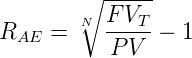## Realized Tax Rate, Effective Capital Gains, and Accrual Equivalent Tax Calculations for L3

In our coverage of FVIF and tax drag we looked at the consequences of applying one tax at a time. But in reality a tax regime will levy taxes across various components of one’s investment returns at different rates. Dividends will be taxed at different rates than capital gains and so on.

In this post we break apart the interaction of these effects to understand the tax rate an investor is actually paying.### Calculating the Realized Tax Rate

The realized tax rate is a weighted average of the taxes you pay on interest, dividends, capital gains.This should be pretty straightforward.

From there, we can take the annualized return after realized taxes. So this is just your return times one minus that weighted average.

The annual return after realized taxes, $R_{ART}$:

$R_{ART}=R \times \left [ 1-P_IT_I-P_DT_D-P_{CG}T_{CG} \right ]$

Which just says that our realized return is the $\text{return} \times (1 - \text{weighted average tax rate})$.

This is an intuitive and important equation to know cold.

### Calculating the Effective Capital Gains Tax

So, the effective capital gains tax basically builds on the blended after-tax return that we just saw but adjusts the capital gains tax rate so that you're not penalized for taxes you've already paid.

In other words the effective capital gains tax $\left ( T_{ECG} \right )$ adjusts the capital gains tax rate gain to reflect previously taxed dividends, income and realized capital gains so that they will not be double taxed. The formula is:

$T_{ECG}=\frac{T_{CG}(1-P_I-P_D-P_{CG})}{(1-P_IT_I-P_DT_D-P_{CG}T_{CG})}$

The equation looks more daunting than it really is.

The numerator is just one minus all individual proportions of returns times the capital gains tax rate. The denominator is one minus the realized tax rate.

You should know that the ratio from this equation is always going to be less than one which means the effective capital gains tax rate is always less than the stated capital gains tax. In summary, this is one of those annoying equations that is a bit in the weeds, won't be worth a ton of points if it comes up, but is still probably worth memorizing.## The Must-Memorize FVIF Equation

Finally we can tie all of these tax equations together to come up with this final FVIF factor in order to calculate how the rate at which we compound our initial assets is a product of (1) our return after realized taxes, (2) the effective capital gains tax rate, and (3) our initial cost basis:

$\text{FVIF}_T=[(1+R_{ART})^n(1-T_{ECG})]+T_{ECG}-(1-B)T_{CG}$

If this comes up on the CFA Level 3 exam it should be fairly plug and play. So just memorize this one.

### Accrual Equivalent Return

Okay. So, I think we've seen that entwining the overall impact of taxes can be kind of difficult and it is easy to get lost in the nuance of each equation.

So let's take a step back and end with a few important summary calculations.

One useful way to summarize the overall impact of taxes is via an accrual equivalent return.

The accrual equivalent return is essentially the tax-free return that produces the same terminal value as the taxable portfolio. If it helps, you can think of it as the effective annual return, or the IRR, that ties together the present value and future value.

The formula is:Alternatively you can use your financial calculator to solve for I/Y by plugging in FV, PV, and N. I/Y represents the geometric average return for the period in question. The difference between the accrual equivalent return and the taxable return is a measure of the tax drag on the portfolio

You can also use your financial calculator by just plugging in those values and solving for I/Y. From an interpretation standpoint I/Y would represent the geometric average return for the period in question.

### Accrual Equivalent Tax Rate

The accrual equivalent tax rate is the rate that explains the difference between tax-free and after-tax returns. In other words,it's the tax rate that makes the pre-tax return equal to the accrual equivalent return (and is therefore another way to measure tax drag).

More directly the accrual equivalent tax rate is the difference between the accrual equivalent return and the taxable return:

$T_{AE}=1-\frac{R_{AE}}{R}$

Where $R_{AE}$ is the accrual equivalent return.

If we think back to our basic tax principles one thing should be obvious even within the endless detail of these calculations.

And that's that the longer you defer realizing capital gains and paying taxes, the higher your accrual equivalent after-tax return will be. So the moral of the story is, don't pay taxes, at least, if you can legally help it (and as an investment advisor help your clients earn tax alpha).

### Taxes and Investment Risk

This is another highly testable concept that's very straightforward.

The basic idea is that when taxes are taken out on an annual basis it reduces investment returns (obviously), but it can also reduce investment risk.

The best way to think about this is that the government is essentially taking on a claim to your returns and they're now essentially an investment partner. with you. Probably an unwelcome one, certainly, but definitely, an investment partner.

From a calculation standpoint he risk that you take is actually reduced by the standard deviation times one minus the tax rate. This is a straightforward, but need to know equation. It has come up on 4-5 CFA Level 3 exams in the past.

Summarizing Investment Risk and Annual TaxesWhat are the implications for investment behavior?

• Hold heavily taxed assets in a tax-advantaged account
• Hold lightly taxed assets in a taxable account
• If you have unrealized losses you’d rather have that in a taxable account so you can offset other gains via tax loss harvesting